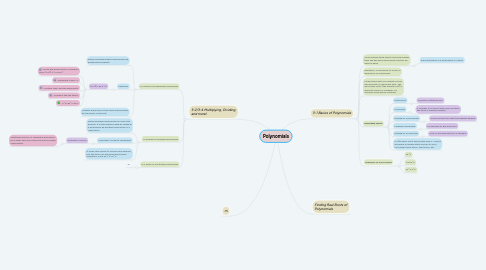# PolynomialsKaitlyn McLane
Get Started. It's FreePolynomials## 1. 3-1 Basics of Polynomials

### 1.1. Some people think about monomials when they see the word polynomial.The two go hand in hand.

1.1.1. Each monomial in a polynomial is a term.

### 1.4. Important Terms

1.4.1. Polynomial

1.4.1.1. Definition stated above.

1.4.2. Monomial

1.4.2.1. A number or product with only one term; the term is a whole number.

1.4.3. Degree of a Polynomial

1.4.3.1. Given by the term with the highest degree.

1.4.4.1. Coefficient of the first term.

1.4.5. Degree of a Monomial

1.4.5.1. Sum of the exponents or a variable.

1.4.6. A little hack: most words that end in -nomial and have a number start such as tri or bi just mean three terms, two terms, etc.

1.5.1. 5x^2

1.5.2. 0.20x^6

1.5.3. 2x^4-x^2

## 2. 3-2/3-4 Multiplying, Dividing, and more!

### 2.1. 3-2 Rules of Multiplying Polynomials

2.1.1. When multiplying two polynomials use distributive property.

2.1.2. Example!

2.1.2.1. (x-2)(1+3x-x^2)

2.1.2.1.1. : Write the polynomials in standard form. (x-2)(-x^2+3x+1)

2.1.2.1.2. : Distribute x then -2.

2.1.2.1.3. : Multiply then add the exponents.

2.1.2.1.4. : Combine the like terms.

2.1.2.1.5. : -x^3+5x^2-5x-2

2.1.3. Multiply each term in the second polynomial by the terms in the first.

### 2.2. 3-3 Rules of Dividing Polynomials

2.2.1. When dividing polynomials you use long division. It is the method used by dividing a polynomial by another polynomial of a lower term.

2.2.2. Important Vocab to remember!

2.2.2.1. Synthetic Division

2.2.2.1.1. Shorthand version of dividing a polynomial by a linear binomial using only the provided coefficients.

2.2.3. It looks very similar to normal long division, but this time you are using the smaller equations, such as x^2-2x^4.

2.3.1. m